RHS Congruency Criteria

Congruence of Triangles
Concept wise

To prove two triangles congruent,

We use RHS criteria when

• R ight angle
• H ypotenuse
• S ide are equal

In RHS congruence criteria,

• Both triangle will have a right angle.
• Hypotenuse of both triangles are equal.
• Anyone of other two sides of both triangle are equal.

For example: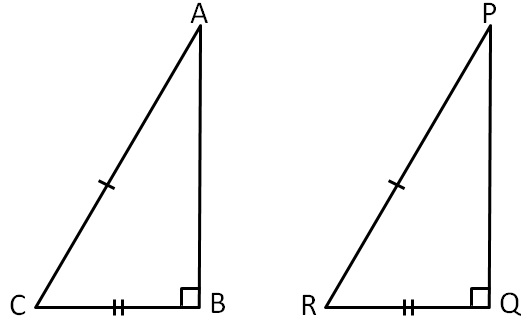Here,

Both of these triangles have

• right angle,
• have their hypotenuse equal
• and another side equal.
•

So, they are congruent

Let’s see more examples

### Are these triangles congruent?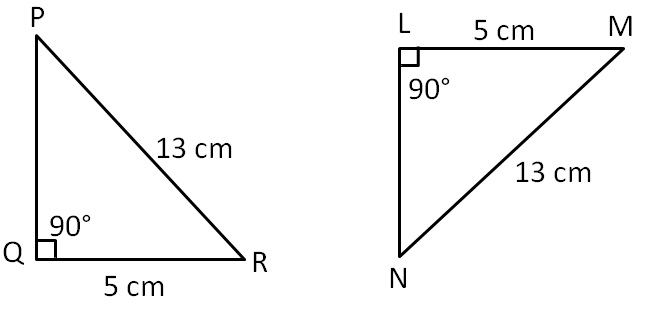In ∆PQR & ∆NLM,

∠Q = ∠L  (    R ight angle, both 90°)

PR = NM    ( H ypotenuse, both 13 cm)

QR = LM    ( S ide, both 5 cm)

∴ ∆PQR ≅ ∆NLM  (RHS Congruence Rule)

### Are these triangles congruent?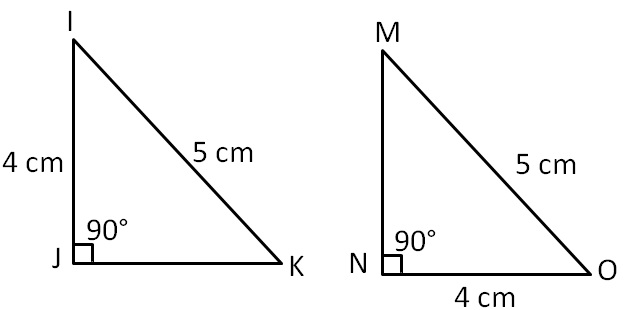In ∆IJK ≅ ∆ONM,

∠J = ∠N     ( R ight angle, both 90°)

IK = OM     ( H ypotenuse, both 5 cm)

IJ = ON        ( S ide, both 4 cm)

∴ ∆IJK ≅ ∆ONM     (RHS Congruence Rule)

### Are these triangles congruent?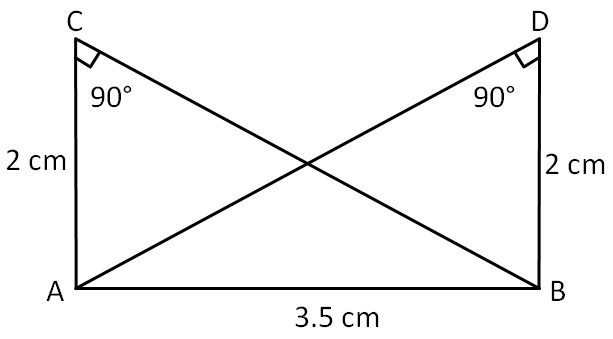∠C = ∠D           ( R ight angle, both 90°)

AB = BA           ( H ypotenuse, common)

AC = BD           ( S ide, both 2 cm)

∴ ∆ABC ≅ ∆BAD       (RHS Congruence Rule)

### Are these triangles congruent?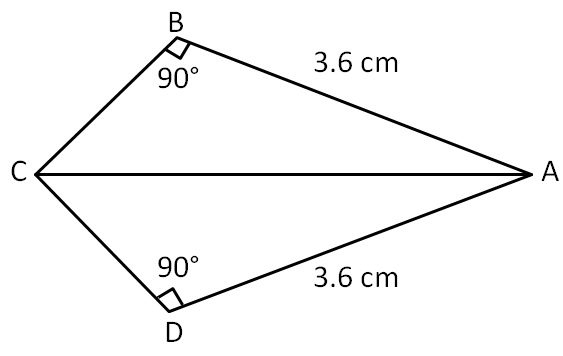∠B = ∠D           ( R ight angle, both 90°)

AC = AC           ( H ypotenuse, common)

AB = AD           ( S ide, both 3.6 cm)

∴ ∆ABC ≅ ∆ADC     (RHS Congruence Rule)

### Are these triangles congruent?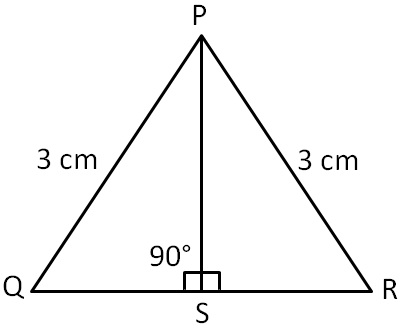In ∆PSQ and ∆PSR,

∠PSQ = ∠PSR            ( R ight angle, both 90°)

PQ = PR                     ( H ypotenuse, both 3 cm)

PS = PS                      ( S ide, common)

∴ ∆PSQ ≅ ∆PSR          (RHS Congruence Rule)

#### (iii) Is ∠DCB = ∠EBC? Why or why not?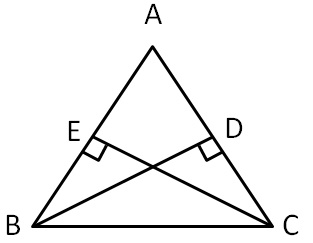(i)In ∆CBD and ∆BCE

∠CDB = ∠BED        ( R ight angle, both 90°)

CB = BC                  ( H ypotenuse, common)

BD = CE                  ( S ide, given)

(ii) ∴ ∆CBD ≅ ∆BCE      (RHS Congruence Rule )

(iii) Now,

∠DCB = ∠EBC             (By CPCT )

#### (iv) Is BD = CD? Why or why not?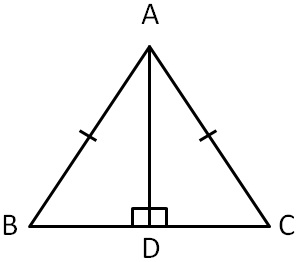AB = AC              ( H ypotenuse, given)

(iii) Now, ∠B = ∠C      (By CPCT )

(iv) Also, BD = CD     (By CPCT)

Learn in your speed, with individual attention - Teachoo Maths 1-on-1 Class